If you're running AdBlock, please consider whitelisting this site if you'd like to support LearnOpenGL; and no worries, I won't be mad if you don't :)

# Skeletal Animation

3D Animations can bring our games to life. Objects in 3D world like humans and & animals feel more organic when they move their limbs to do certain things like walking, running & attacking. This tutorial is about Skeletal animation which you all have been waiting for. We will first understand the concept thoroughly and then understand the data we need to animate a 3D model using Assimp. I'd recommend you to finish the Model Loading chapter of this saga as this tutorial code continues from there. You can still understand the concept and implement it in your way. So let's get started.

### Interpolation

To understand how animation works at basic level we need to understand the concept of Interpolation. Interpolation can be defined as something happening over time. Like an enemy moving from point A to point B in time T i.e Translation happening over time . A gun turret smoothly rotates to face the target i.e Rotation happening over time and a tree is scaling up from size A to size B in time T i.e Scaling happening over time.

A simple interpolation equation used for Translation and Scale looks like this..

a = a * (1 - t) + b * t

It is known as as Linear Interpolation equation or Lerp. For Rotation we cannot use Vector. The reason for that is if we went ahead and tried to use the linear interpolation equation on a vector of X(Pitch),Y(Yaw) & Z(Roll), the interpolation won't be linear. You will encounter weird issues like The Gimbal Lock(See references section below to learn about it). To avoid this issue we use Quaternion for rotations. Quaternion provides something called The Spherical Interpolation or Slerp equation which gives the same result as Lerp but for two rotations A & B. I won't be able to explain how the equation works because its out of the scope for now. You can surely checkout references section below to understand The Quaternion.

### Components of An Animated Model : Skin, Bones and Keyframes

The whole process of an animation starts with the addition of the first component which is The Skin in a software like blender or Maya. Skin is nothing but meshes which add visual aspect to the model to tell the viewer how it looks like. But If you want to move any mesh then just like the real world, you need to add Bones. You can see the images below to understand how it looks in software like blender....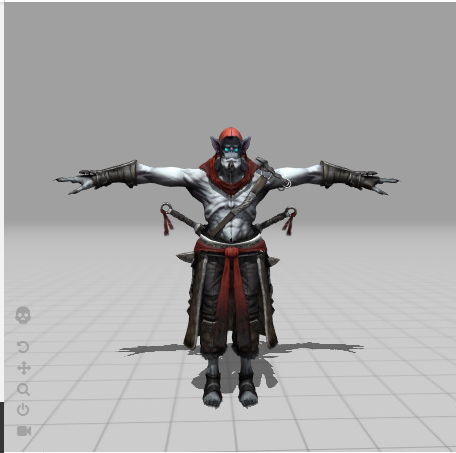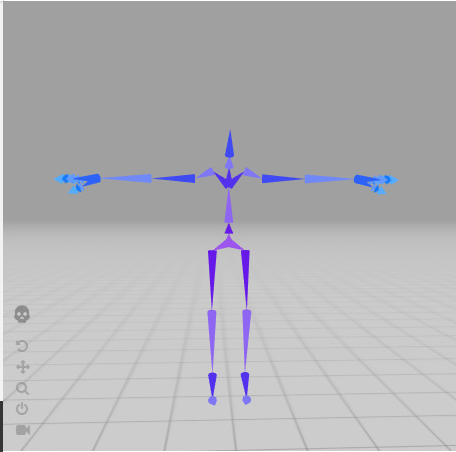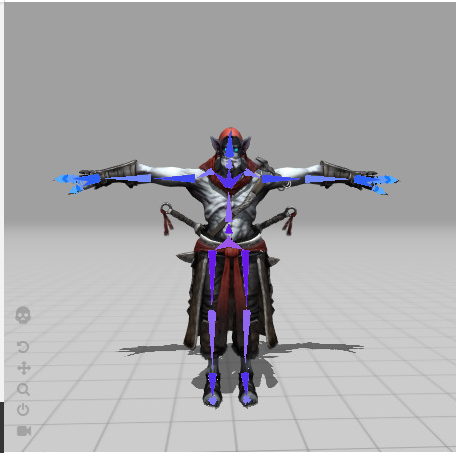These bones are usually added in hierarchical fashion for characters like humans & animals and the reason is pretty obvious. We want parent-child relationship among limbs. For example, If we move our right shoulder then our right bicep, forearm, hand and fingers should move as well. This is how the hierarchy looks like....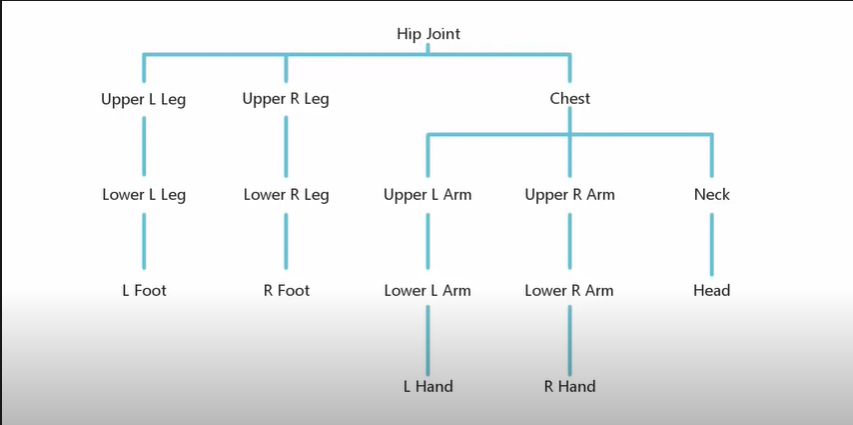In the above diagram if you grab the hip bone and move it, all limbs will be affected by its movement.

At this point, we are ready to create KeyFrames for an animation. Keyframes are poses at different point of time in an animation. We will interpolate between these Keyframes to go from one pose to another pose smoothly in our code. Below you can see how poses are created for a simple 4 frame jump animation...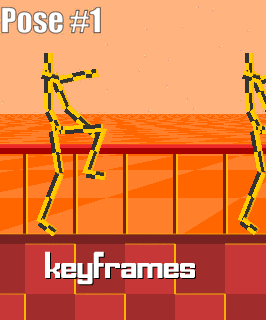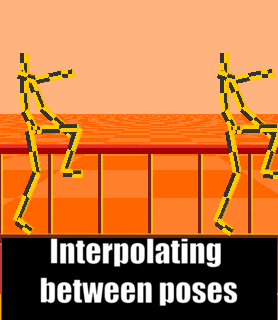### How Assimp holds animation data

We are almost there to the code part but first we need to understand how assimp holds imported animation data. Look at the diagram below..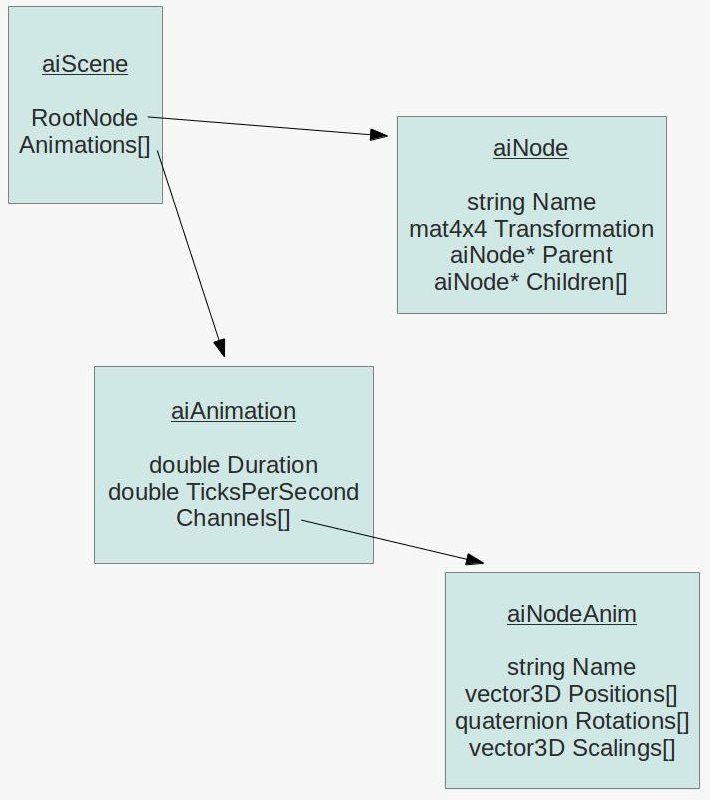Just like in the Model Loading chapter, we will start with the aiScene pointer which holds a pointer to the root node and look what do we have here, an array of Animations. This array of aiAnimation contains the general information like duration of an animation represented here as mDuration and then we have a mTicksPerSecond variable, which controls how fast we should interpolate between frames. If you remember from the last section that an animation has keyframes. Similary, an aiAnimation contains an aiNodeAnim array called Channels. This array of contains all bones and their keyframes which are going to be engaged in an animation. An aiNodeAnim contains name of the bone and you will find 3 types of keys to interpolate between here, Translation,Rotation & Scale.

Alright, there's one last thing we need to understand and we are good to go for writing some code.

### Influence of multiple bones on vertices

When we curl our forearm and we see our biceps muscle pop up. We can also say that forearm bone transformation is affecting vertices on our biceps. Similary, there could be multiple bones affecting a single vertex in a mesh. For characters like solid metal robots all forearm vertices will only be affected by forearm bone but for characters like humans, animals etc, there could be upto 4 bones which can affect a vertex.  Let's see how assimp stores that information...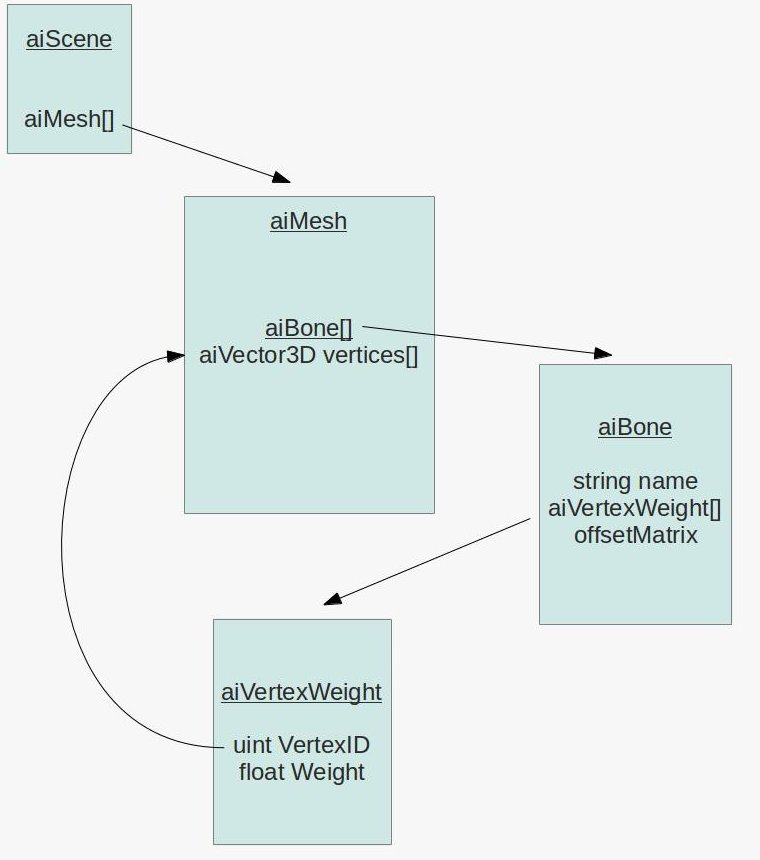We start with the aiScene pointer again which contains an array of all aiMeshes. Each aiMesh object has an array of aiBone which contains the information like how much influence this aiBone will have on set of vertices on the mesh. aiBone contains the name of the bone, an array of aiVertexWeight which basically tells us how much influence this aiBone will have on what vertices on the mesh. Now we have one more member of aiBone which is offsetMatrix. It's a 4x4 matrix used to transform vertices from model space to their bone space. You can see this in action in images below....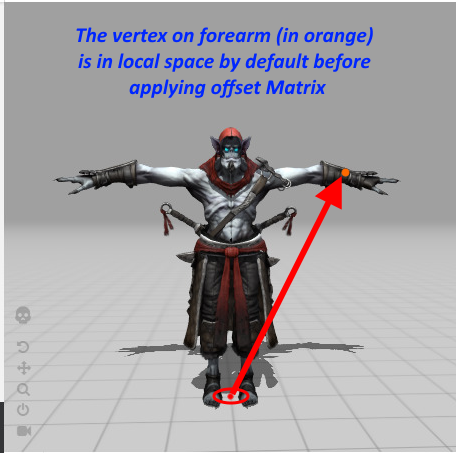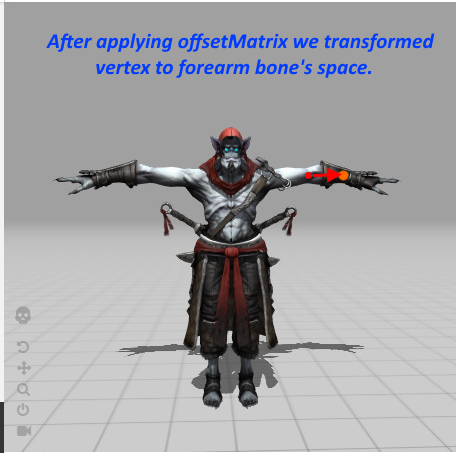When vertices are in bone space they will be transformed relative to their bone as they are supposed to. You will soon see this in action in code.

### Finally! Let's code.

Thank you for making it this far. We will start with directly looking at the end result which is our final vertex shader code. This will give us good sense what we need at the end..

#version 430 core

layout(location = 0) in vec3 pos;
layout(location = 1) in vec3 norm;
layout(location = 2) in vec2 tex;
layout(location = 3) in ivec4 boneIds;
layout(location = 4) in vec4 weights;

uniform mat4 projection;
uniform mat4 view;
uniform mat4 model;

const int MAX_BONES = 100;
const int MAX_BONE_INFLUENCE = 4;
uniform mat4 finalBonesMatrices[MAX_BONES];

out vec2 TexCoords;

void main()
{
vec4 totalPosition = vec4(0.0f);
for(int i = 0 ; i < MAX_BONE_INFLUENCE ; i++)
{
if(boneIds[i] == -1)
continue;
if(boneIds[i] >= MAX_JOINTS)
{
totalPosition = vec4(pos,1.0f);
break;
}
vec4 localPosition = finalBoneMatrices[boneIds[i]] * vec4(pos,1.0f);
totalPosition += localPosition * weights[i];
vec3 localNormal = mat3(finalBoneMatrices[boneIds[i]]) * norm;
}

mat4 viewModel = view * model;
gl_Position =  projection * viewModel * totalPosition;
TexCoords = tex;
}


Fragment shader remains the same from the model loading chapter. Starting from the top you see two new attributes layout declaration. First boneIds and second is weights. we also have a uniform array finalBonesMatrices which stores transformations of all bones.   boneIds contains indices which are used to read the finalBonesMatrices array and apply those transformation to pos vertex with their respective weights stored in  weights  array. This happens inside  for  loop above. Now let's add support in our Mesh class for bone weights first..

#define MAX_BONE_INFLUENCE 4

struct Vertex {
// position
glm::vec3 Position;
// normal
glm::vec3 Normal;
// texCoords
glm::vec2 TexCoords;

//bone indexes which will influence this vertex
int m_BoneIDs[MAX_BONE_INFLUENCE];

//weights from each bone
float m_Weights[MAX_BONE_INFLUENCE];
};


We have added two new attributes for the Vertex, just like we saw in our vertex shader. Now's let's load them in GPU buffers just like other attributes in our Mesh::setupMesh  function...

class Mesh
{
...

void setupMesh()
{
...

// ids
glEnableVertexAttribArray(3);
glVertexAttribIPointer(3, 4, GL_INT, sizeof(Vertex),
(void*)offsetof(Vertex, m_BoneIDs));

// weights
glEnableVertexAttribArray(4);
glVertexAttribPointer(4, 4, GL_FLOAT, GL_FALSE, sizeof(Vertex),
(void*)offsetof(Vertex, m_Weights));

...
}
...
}


Just like before, except now we have added 3 and 4 layout location ids for boneIds and weights. One imporant thing to notice here is how we are passing data for boneIds. We are using glVertexAttribIPointer and we passed GL_INT as third parameter.

Now we can extract the bone-weight information from the assimp data structure. Let's make some changes in Model class...

struct BoneInfo
{
/*id is index in finalBoneMatrices*/
int id;

/*offset matrix transforms vertex from model space to bone space*/
glm::mat4 offset;

};


This  BoneInfo  will store our offset matrix and also a unique id which will be used as an index to store it in finalBoneMatrices array we saw earlier in our shader. Now we will add bone weight extraction support in Model...

class Model
{
private:
...
std::map<string, BoneInfo> m_BoneInfoMap; //
int m_BoneCounter = 0;

...
void SetVertexBoneDataToDefault(Vertex& vertex)
{
for (int i = 0; i < MAX_BONE_WEIGHTS; i++)
{
vertex.m_BoneIDs[i] = -1;
vertex.m_Weights[i] = 0.0f;
}
}

Mesh processMesh(aiMesh* mesh, const aiScene* scene)
{
vector vertices;
vector indices;
vector textures;

for (unsigned int i = 0; i < mesh->mNumVertices; i++)
{
Vertex vertex;

SetVertexBoneDataToDefault(vertex);

vertex.Position = AssimpGLMHelpers::GetGLMVec(mesh->mVertices[i]);
vertex.Normal = AssimpGLMHelpers::GetGLMVec(mesh->mNormals[i]);

if (mesh->mTextureCoords)
{
glm::vec2 vec;
vec.x = mesh->mTextureCoords[i].x;
vec.y = mesh->mTextureCoords[i].y;
vertex.TexCoords = vec;
}
else
vertex.TexCoords = glm::vec2(0.0f, 0.0f);

vertices.push_back(vertex);
}
...
ExtractBoneWeightForVertices(vertices,mesh,scene);

return Mesh(vertices, indices, textures);
}

void SetVertexBoneData(Vertex& vertex, int boneID, float weight)
{
for (int i = 0; i < MAX_BONE_WEIGHTS; ++i)
{
if (vertex.m_BoneIDs[i] < 0)
{
vertex.m_Weights[i] = weight;
vertex.m_BoneIDs[i] = boneID;
break;
}
}
}

void ExtractBoneWeightForVertices(std::vector& vertices, aiMesh* mesh,
const aiScene* scene)
{
for (int boneIndex = 0; boneIndex < mesh->mNumBones; ++boneIndex)
{
int boneID = -1;
std::string boneName = mesh->mBones[boneIndex]->mName.C_Str();
if (m_BoneInfoMap.find(boneName) == m_BoneInfoMap.end())
{
BoneInfo newBoneInfo;
newBoneInfo.id = m_BoneCounter;
newBoneInfo.offset = AssimpGLMHelpers::
ConvertMatrixToGLMFormat(mesh->mBones[boneIndex]->mOffsetMatrix);
m_BoneInfoMap[boneName] = newBoneInfo;
boneID = m_BoneCounter;
m_BoneCounter++;
}
else
{
boneID = m_BoneInfoMap[boneName].id;
}
assert(boneID != -1);
auto weights = mesh->mBones[boneIndex]->mWeights;
int numWeights = mesh->mBones[boneIndex]->mNumWeights;

for (int weightIndex = 0; weightIndex < numWeights; ++weightIndex)
{
int vertexId = weights[weightIndex].mVertexId;
float weight = weights[weightIndex].mWeight;
assert(vertexId <= vertices.size());
SetVertexBoneData(vertices[vertexId], boneID, weight);
}
}
}
.......
};


We start by declaring a map m_BoneInfoMap and a counter m_BoneCounter which will be incremented as soon as we read a new bone. we saw in the diagram earlier that each aiMesh contains all aiBones which are associated with the aiMesh. The whole process of the bone-weight extraction starts from the  processMesh  function. For each loop iteration we are setting m_BoneIDs and m_Weights to their default values by calling function SetVertexBoneDataToDefault. Just before the processMesh function ends, we call the ExtractBoneWeightData. In the ExtractBoneWeightData we run a for loop for each aiBone and check if this bone already exists in the m_BoneInfoMap. If we couldn't find it then it's considered a new bone and we create new BoneInfo with an id and store its associated mOffsetMatrix to it. Then we store this new BoneInfo in m_BoneInfoMap and then we increment the m_BoneCounter counter to create an id for next bone. In case we find the bone name in m_BoneInfoMap then that means this bone affects vertices of mesh out of its scope. So we take it's Id and proceed further to know which vertices it affects.

One thing to notice that we are calling AssimpGLMHelpers::ConvertMatrixToGLMFormat. Assimp store its matrix data in different format than GLM so this function just gives us our matrix in GLM format.

We have extracted the offsetMatrix for the bone and now we will simply iterate its aiVertexWeightarray and extract all vertices indices which will be influenced by this bone along with their respective weights and call SetVertexBoneData to fill up Vertex.boneIds and Vertex.weights with extracted information.

Phew! You deserve a coffee break at this point.

### Bone,Animation & Animator classes

Here's high level view of classes..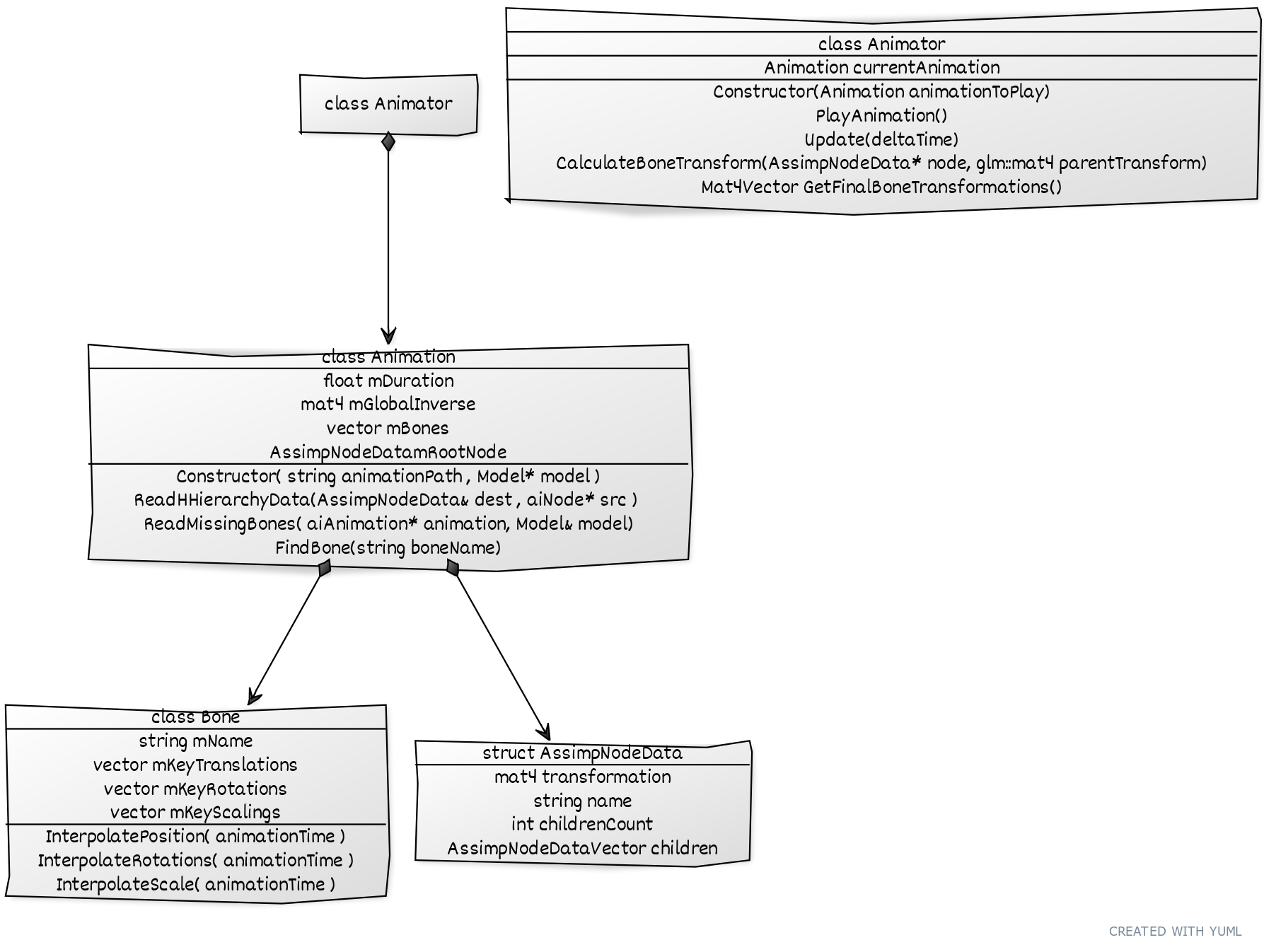Let us remind ourselves what we are trying to achieve. For each rendering frame we want to interpolate all bones in heirarchy smoothly and get their final transformations matrices which will be supplied to shader uniform finalBonesMatrices. Here's what each class does...

Bone : A single bone which reads all keyframes data from aiNodeAnim. It will also interpolate between its keys i.e Translation,Scale & Rotation based on the current animation time.

AssimpNodeData : This struct will help us to isolate our Animation from Assimp.

Animation : An asset which reads data from aiAnimation and create a heirarchical record of Bones

Animator : This will read the heirarchy of AssimpNodeData, Interpolate all bones in a recursive manner and then prepare final bone transformation matrices for us that we need.

Here's the code for Bone...

struct KeyPosition
{
glm::vec3 position;
float timeStamp;
};

struct KeyRotation
{
glm::quat orientation;
float timeStamp;
};

struct KeyScale
{
glm::vec3 scale;
float timeStamp;
};

class Bone
{
private:
std::vector<KeyPosition> m_Positions;
std::vector<KeyRotation> m_Rotations;
std::vector<KeyScale> m_Scales;
int m_NumPositions;
int m_NumRotations;
int m_NumScalings;

glm::mat4 m_LocalTransform;
std::string m_Name;
int m_ID;
public:
/*reads keyframes from aiNodeAnim*/
Bone(const std::string& name, int ID, const aiNodeAnim* channel)
:
m_Name(name),
m_ID(ID),
m_LocalTransform(1.0f)
{
m_NumPositions = channel->mNumPositionKeys;

for (int positionIndex = 0; positionIndex < m_NumPositions; ++positionIndex)
{
aiVector3D aiPosition = channel->mPositionKeys[positionIndex].mValue;
float timeStamp = channel->mPositionKeys[positionIndex].mTime;
KeyPosition data;
data.position = AssimpGLMHelpers::GetGLMVec(aiPosition);
data.timeStamp = timeStamp;
m_Positions.push_back(data);
}

m_NumRotations = channel->mNumRotationKeys;
for (int rotationIndex = 0; rotationIndex < m_NumRotations; ++rotationIndex)
{
aiQuaternion aiOrientation = channel->mRotationKeys[rotationIndex].mValue;
float timeStamp = channel->mRotationKeys[rotationIndex].mTime;
KeyRotation data;
data.orientation = AssimpGLMHelpers::GetGLMQuat(aiOrientation);
data.timeStamp = timeStamp;
m_Rotations.push_back(data);
}

m_NumScalings = channel->mNumScalingKeys;
for (int keyIndex = 0; keyIndex < m_NumScalings; ++keyIndex)
{
aiVector3D scale = channel->mScalingKeys[keyIndex].mValue;
float timeStamp = channel->mScalingKeys[keyIndex].mTime;
KeyScale data;
data.scale = AssimpGLMHelpers::GetGLMVec(scale);
data.timeStamp = timeStamp;
m_Scales.push_back(data);
}
}

/* Interpolates b/w positions,rotations & scaling keys based on the curren time of the
animation and prepares the local transformation matrix by combining all keys tranformations */
void Update(float animationTime)
{
glm::mat4 translation = InterpolatePosition(animationTime);
glm::mat4 rotation = InterpolateRotation(animationTime);
glm::mat4 scale = InterpolateScaling(animationTime);
m_LocalTransform = translation * rotation * scale;
}

glm::mat4 GetLocalTransform() { return m_LocalTransform; }
std::string GetBoneName() const { return m_Name; }
int GetBoneID() { return m_ID; }

/* Gets the current index on mKeyPositions to interpolate to based on the current
animation time */
int GetPositionIndex(float animationTime)
{
for (int index = 0; index < m_NumPositions - 1; ++index)
{
if (animationTime < m_Positions[index + 1].timeStamp)
return index;
}
assert(0);
}

/* Gets the current index on mKeyRotations to interpolate to based on the current
animation time */
int GetRotationIndex(float animationTime)
{
for (int index = 0; index < m_NumRotations - 1; ++index)
{
if (animationTime < m_Rotations[index + 1].timeStamp)
return index;
}
assert(0);
}

/* Gets the current index on mKeyScalings to interpolate to based on the current
animation time */
int GetScaleIndex(float animationTime)
{
for (int index = 0; index < m_NumScalings - 1; ++index)
{
if (animationTime < m_Scales[index + 1].timeStamp)
return index;
}
assert(0);
}
private:

/* Gets normalized value for Lerp & Slerp*/
float GetScaleFactor(float lastTimeStamp, float nextTimeStamp, float animationTime)
{
float scaleFactor = 0.0f;
float midWayLength = animationTime - lastTimeStamp;
float framesDiff = nextTimeStamp - lastTimeStamp;
scaleFactor = midWayLength / framesDiff;
return scaleFactor;
}

/* figures out which position keys to interpolate b/w and performs the interpolation
and returns the translation matrix */
glm::mat4 InterpolatePosition(float animationTime)
{
if (1 == m_NumPositions)
return glm::translate(glm::mat4(1.0f), m_Positions.position);

int p0Index = GetPositionIndex(animationTime);
int p1Index = p0Index + 1;
float scaleFactor = GetScaleFactor(m_Positions[p0Index].timeStamp,
m_Positions[p1Index].timeStamp, animationTime);
glm::vec3 finalPosition = glm::mix(m_Positions[p0Index].position,
m_Positions[p1Index].position
, scaleFactor);
return glm::translate(glm::mat4(1.0f), finalPosition);
}

/* figures out which rotations keys to interpolate b/w and performs the interpolation
and returns the rotation matrix */
glm::mat4 InterpolateRotation(float animationTime)
{
if (1 == m_NumRotations)
{
auto rotation = glm::normalize(m_Rotations.orientation);
return glm::toMat4(rotation);
}

int p0Index = GetRotationIndex(animationTime);
int p1Index = p0Index + 1;
float scaleFactor = GetScaleFactor(m_Rotations[p0Index].timeStamp,
m_Rotations[p1Index].timeStamp, animationTime);
glm::quat finalRotation = glm::slerp(m_Rotations[p0Index].orientation,
m_Rotations[p1Index].orientation, scaleFactor);
finalRotation = glm::normalize(finalRotation);
return glm::toMat4(finalRotation);
}

/* figures out which scaling keys to interpolate b/w and performs the interpolation
and returns the scale matrix */
glm::mat4 Bone::InterpolateScaling(float animationTime)
{
if (1 == m_NumScalings)
return glm::scale(glm::mat4(1.0f), m_Scales.scale);

int p0Index = GetScaleIndex(animationTime);
int p1Index = p0Index + 1;
float scaleFactor = GetScaleFactor(m_Scales[p0Index].timeStamp,
m_Scales[p1Index].timeStamp, animationTime);
glm::vec3 finalScale = glm::mix(m_Scales[p0Index].scale,
m_Scales[p1Index].scale, scaleFactor);
return glm::scale(glm::mat4(1.0f), finalScale);
}
};


We start by creating 3 structs for our key types. Each struct holds a value and a time stamp. Timestamp tells us at what point of an animation we need to interpolate to its value. Bone has a constructor which reads from aiNodeAnim and stores keys and their timestamps to mPositionKeys, mRotationKeys & mScalingKeys . The main interpolation process starts from Update(float animationTime) which gets called every frame. This function calls respective interpolation functions for all key types and combines all final interpolation results and store it to a 4x4 Matrix m_LocalTransform. The interpolations functions for translation & scale keys are similar but for rotation we are using Slerp to interpolate between quaternions. Both Lerp & Slerp takes 3 arguments. First argument takes last key, second argument takes next key and third argument takes value of range 0-1,we call it scale factor here. Let's see how we calculate this scale factor in function GetScaleFactor...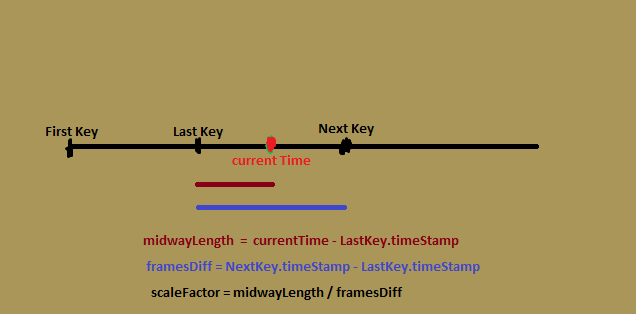In code...

float midWayLength = animationTime - lastTimeStamp;

float framesDiff = nextTimeStamp - lastTimeStamp;

scaleFactor = midWayLength / framesDiff;

Let's move on to Animation class now...
struct AssimpNodeData
{
glm::mat4 transformation;
std::string name;
int childrenCount;
std::vector<AssimpNodeData> children;
};

class Animation
{
public:
Animation() = default;

Animation(const std::string& animationPath, Model* model)
{
Assimp::Importer importer;
const aiScene* scene = importer.ReadFile(animationPath, aiProcess_Triangulate);
assert(scene && scene->mRootNode);
auto animation = scene->mAnimations;
m_Duration = animation->mDuration;
m_TicksPerSecond = animation->mTicksPerSecond;
ReadHeirarchyData(m_RootNode, scene->mRootNode);
ReadMissingBones(animation, *model);
}

~Animation()
{
}

Bone* FindBone(const std::string& name)
{
auto iter = std::find_if(m_Bones.begin(), m_Bones.end(),
[&](const Bone& Bone)
{
return Bone.GetBoneName() == name;
}
);
if (iter == m_Bones.end()) return nullptr;
else return &(*iter);
}

inline float GetTicksPerSecond() { return m_TicksPerSecond; }

inline float GetDuration() { return m_Duration;}

inline const AssimpNodeData& GetRootNode() { return m_RootNode; }

inline const std::map<std::string,BoneInfo>& GetBoneIDMap()
{
return m_BoneInfoMap;
}

private:
void ReadMissingBones(const aiAnimation* animation, Model& model)
{
int size = animation->mNumChannels;

auto& boneInfoMap = model.GetBoneInfoMap();//getting m_BoneInfoMap from Model class
int& boneCount = model.GetBoneCount(); //getting the m_BoneCounter from Model class

//reading channels(bones engaged in an animation and their keyframes)
for (int i = 0; i < size; i++)
{
auto channel = animation->mChannels[i];
std::string boneName = channel->mNodeName.data;

if (boneInfoMap.find(boneName) == boneInfoMap.end())
{
boneInfoMap[boneName].id = boneCount;
boneCount++;
}
m_Bones.push_back(Bone(channel->mNodeName.data,
boneInfoMap[channel->mNodeName.data].id, channel));
}

m_BoneInfoMap = boneInfoMap;
}

void ReadHeirarchyData(AssimpNodeData& dest, const aiNode* src)
{
assert(src);

dest.name = src->mName.data;
dest.transformation = AssimpGLMHelpers::ConvertMatrixToGLMFormat(src->mTransformation);
dest.childrenCount = src->mNumChildren;

for (int i = 0; i < src->mNumChildren; i++)
{
AssimpNodeData newData;
ReadHeirarchyData(newData, src->mChildren[i]);
dest.children.push_back(newData);
}
}
float m_Duration;
int m_TicksPerSecond;
std::vector<Bone> m_Bones;
AssimpNodeData m_RootNode;
std::map<std::string, BoneInfo> m_BoneInfoMap;
};


Here, creation of an Animation object starts with a constructor. It takes two arguments. First, path to the animation file & second parameter is the Model for this animation. You will see later ahead why we need this Model reference here. We then create an Assimp::Importer to read the animation file, followed by an assert check which will throw an error if animation could not be found. Then we read general animation data like how long is this animation which is mDuration and the animation speed represented by mTicksPerSecond. We then call ReadHeirarchyData which replicates aiNode heirarchy of Assimp and creates heirarchy of AssimpNodeData.

Then we call a function called ReadMissingBones. I had to write this function because sometimes when I loaded FBX model separately, it had some bones missing and I found those missing bones in the animation file. This function reads the missing bones information and stores their information in m_BoneInfoMap of Model and saves a reference of m_BoneInfoMap locally in the m_BoneInfoMap.

And we have our animation ready. Now let's look at our final stage, The Animator class...

class Animator
{
public:
Animator::Animator(Animation* Animation)
{
m_CurrentTime = 0.0;
m_CurrentAnimation = currentAnimation;

m_FinalBoneMatrices.reserve(100);

for (int i = 0; i < 100; i++)
m_FinalBoneMatrices.push_back(glm::mat4(1.0f));
}

void Animator::UpdateAnimation(float dt)
{
m_DeltaTime = dt;
if (m_CurrentAnimation)
{
m_CurrentTime += m_CurrentAnimation->GetTicksPerSecond() * dt;
m_CurrentTime = fmod(m_CurrentTime, m_CurrentAnimation->GetDuration());
CalculateBoneTransform(&m_CurrentAnimation->GetRootNode(), glm::mat4(1.0f));
}
}

void Animator::PlayAnimation(Animation* pAnimation)
{
m_CurrentAnimation = pAnimation;
m_CurrentTime = 0.0f;
}

void Animator::CalculateBoneTransform(const AssimpNodeData* node, glm::mat4 parentTransform)
{
std::string nodeName = node->name;
glm::mat4 nodeTransform = node->transformation;

Bone* Bone = m_CurrentAnimation->FindBone(nodeName);

if (Bone)
{
Bone->Update(m_CurrentTime);
nodeTransform = Bone->GetLocalTransform();
}

glm::mat4 globalTransformation = parentTransform * nodeTransform;

auto boneInfoMap = m_CurrentAnimation->GetBoneIDMap();
if (boneInfoMap.find(nodeName) != boneInfoMap.end())
{
int index = boneInfoMap[nodeName].id;
glm::mat4 offset = boneInfoMap[nodeName].offset;
m_FinalBoneMatrices[index] = globalTransformation * offset;
}

for (int i = 0; i < node->childrenCount; i++)
CalculateBoneTransform(&node->children[i], globalTransformation);
}

std::vector<glm::mat4> GetFinalBoneMatrices()
{
return m_FinalBoneMatrices;
}

private:
std::vector<glm::mat4> m_FinalBoneMatrices;
Animation* m_CurrentAnimation;
float m_CurrentTime;
float m_DeltaTime;
};


Animator constructor takes an animation to play and then it proceeds to reset the animation time m_CurrentTime to 0. It also initializes m_FinalBoneMatrices which is a std::vector<glm::mat4>. The main point of attention here is UpdateAnimation(float deltaTime) function. It advances the m_CurrentTime with rate of m_TicksPerSecond and then calls the CalculateBoneTransform function. We will pass two arguments in the start, first is the m_RootNode of m_CurrentAnimation and second is an identity matrix passed as parentTransform This function then check if m_RootNodes bone is engaged in this animation by finding it in m_Bones array of Animation. If bone is found then it calls Bone.Update() function which interpolates all bones and return local bone transform matrix to nodeTransform. But this is local space matrix and will move bone around origin if passed in shaders. So we multiply this nodeTransform with parentTransform and we store the result in globalTransformation. This would be enough but vertices are still in default model space. we find offset matrix in m_BoneInfoMap and then multiply it with globalTransfromMatrix. We will also get the id index which will be used to write final transformation of this bone to m_FinalBoneMatrices.

Finally! we call CalculateBoneTransform for each child nodes of this node and pass globalTransformation as parentTransform. We break this recursive loop when there will no children left to process further.

### Let's Animate

Fruit of our hardwork is finally here! Here's how we will play the animation in main.cpp ...

int main()
{
...

Model ourModel(FileSystem::getPath("resources/objects/vampire/dancing_vampire.dae"));
Animation danceAnimation(FileSystem::getPath(
"resources/objects/vampire/dancing_vampire.dae"), &ourModel);
Animator animator(&danceAnimation);

// draw in wireframe
//glPolygonMode(GL_FRONT_AND_BACK, GL_LINE);

// render loop
// -----------
while (!glfwWindowShouldClose(window))
{
// per-frame time logic
// --------------------
float currentFrame = glfwGetTime();
deltaTime = currentFrame - lastFrame;
lastFrame = currentFrame;

// input
// -----
processInput(window);
animator.UpdateAnimation(deltaTime);

// render
// ------
glClearColor(0.05f, 0.05f, 0.05f, 1.0f);
glClear(GL_COLOR_BUFFER_BIT | GL_DEPTH_BUFFER_BIT);

// don't forget to enable shader before setting uniforms
ourShader.use();

// view/projection transformations
glm::mat4 projection = glm::perspective(glm::radians(camera.Zoom),
(float)SCR_WIDTH / (float)SCR_HEIGHT, 0.1f, 100.0f);
glm::mat4 view = camera.GetViewMatrix();
ourShader.setMat4("projection", projection);
ourShader.setMat4("view", view);

auto transforms = animator.GetFinalBoneMatrices();
for (int i = 0; i < transforms.size(); ++i)
ourShader.setMat4("finalBonesTransformations[" + std::to_string(i) + "]",
transforms[i]);

// render the loaded model
glm::mat4 model = glm::mat4(1.0f);
model = glm::translate(model, glm::vec3(0.0f, -0.4f, 0.0f));
model = glm::scale(model, glm::vec3(.5f, .5f, .5f));
ourShader.setMat4("model", model);
ourModel.Draw(ourShader);

// glfw: swap buffers and poll IO events (keys pressed/released, mouse moved etc.)
// -------------------------------------------------------------------------------
glfwSwapBuffers(window);
glfwPollEvents();
}

// glfw: terminate, clearing all previously allocated GLFW resources.
// ------------------------------------------------------------------
glfwTerminate();
return 0;
}


We start with loading our Model which will setup bone weight data for the shader and then create an Animation by giving it the path. Then we create our Animator object by passing it the created Animation. In render loop we then update our Animator, take the final bone transformations and give it to shaders. Here's the output we all have been waiting for...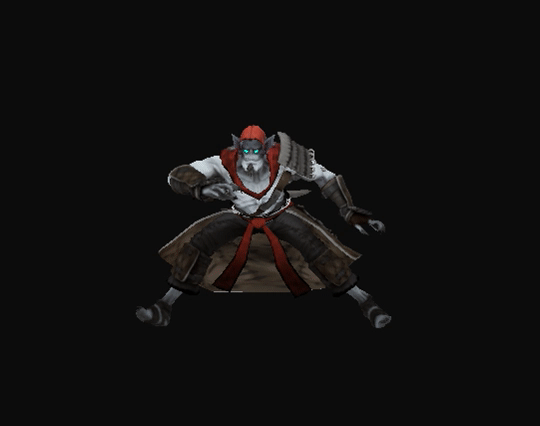Download the model used here. Note that animations and meshes are baked in single DAE(collada) file. You can find the full source code here

### Further reading

Article by: Ankit Singh Kushwah
Contact: e-mail
HI